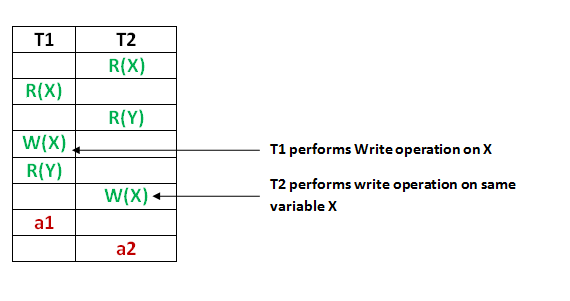# GATE | GATE-CS-2016 (Set 2) | Question 61

Consider the following database schedule with two transactions, T1 and T2.

`S = r2(X); r1(X); r2(Y); w1(X); r1(Y); w2(X); a1; a2;`

where ri(Z) denotes a read operation by transaction Ti on a variable Z, wi(Z) denotes a write operation by Ti on a variable Z and ai denotes an abort by transaction Ti .
Which one of the following statements about the above schedule is TRUE?
(A) S is non-recoverable
(B) S is recoverable, but has a cascading abort
(C) S does not have a cascading abort

(D) S is strict

Explanation:As we can see in figure,

• T2 overwrites a value that T1 writes
• T1 aborts: its “remembered” values are restored.
• Cascading Abort could have arised if – > Abort of T1 required abort of T2 but as T2 is already aborted , its not a cascade abort. Therefore, Option C

Option A – is not true because the given schedule is recoverable

Option B – is not true as it is recoverable and avoid cascading aborts;

Option D – is not true because T2 is also doing abort operation after T1 does, so NOT strict.

Quiz of this Question